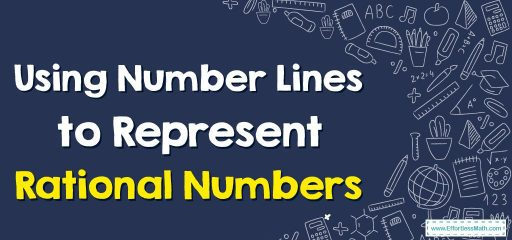# Using Number Lines to Represent Rational NumbersA number line is a visual representation of numbers that are arranged in a linear fashion, where each point on the line corresponds to a specific number. Number lines can be used to represent rational numbers, which are numbers that can be expressed as the ratio of two integers (such as 3/4 or -5/2).

## Step-by-Step Guide to Using Number Lines to Represent Rational Numbers

To use a number line to represent rational numbers, you can follow these steps:

1. Draw a number line: Start by drawing a horizontal line with arrows at both ends to indicate that the numbers continue indefinitely in both directions.
2. Label the number line: Mark the integers (whole numbers) on the number line at equal intervals. Start with zero in the middle and move towards the right for positive numbers, and towards the left for negative numbers.
3. Represent rational numbers: To represent rational numbers on the number line, you can use a point or a tick mark to indicate a specific number. For example, to represent the rational number 3/4, you can place a tick mark at the point on the number line that corresponds to 0.75 (which is 3/4 of the distance between 0 and 1).
4. Represent negative rational numbers: To represent negative rational numbers, you can use a point or tick mark on the left side of the number line, corresponding to the negative value of the number. For example, to represent -3/4, you can place a tick mark at the point on the number line that corresponds to -0.75 (which is 3/4 of the distance between 0 and -1).
5. Use different colors or symbols: To distinguish between different rational numbers on the number line, you can use different colors or symbols (such as circles, squares, etc.) to represent different types of numbers. For example, you can use one color or symbol to represent positive rational numbers and another color or symbol to represent negative rational numbers.
1. Use fractions: You can also use fractions to represent rational numbers on the number line. For example, if you want to represent the rational number 3/4 on the number line, you can use the fraction 3/4 to indicate that the point on the number line is 3/4 of the distance between 0 and 1.
2. Use decimals: Another way to represent rational numbers on the number line is by using decimals. For example, 0.75 on the number line represents 3/4.

Note: Not all rational numbers can be represented on the number line, as there are infinite rational numbers, and it’s impossible to mark all of them on the number line. But this representation can be useful to give a visual representation of the rational numbers and compare them with each other.

### Using Number Lines to Represent Rational Numbers – Examples 1

Graph $$\frac{3}{10}$$ on the number line.

Solution:
Step 1:
Divide the unit length between 0 and 1 by 10 (denominator).

Using Number Lines to Represent Rational Numbers – Examples 2

Graph $$-2.75$$ on the number line.
Solution:
Step 1: Jump two numbers to the left.
Step 2: Divide the unit length between $$-2$$ and $$-3$$ into 4 parts.
Step 3: Count 3 parts and mark it as $$-2.75$$.

### What people say about "Using Number Lines to Represent Rational Numbers - Effortless Math: We Help Students Learn to LOVE Mathematics"?

No one replied yet.

X
30% OFF

Limited time only!

Save Over 30%

SAVE $5 It was$16.99 now it is \$11.99